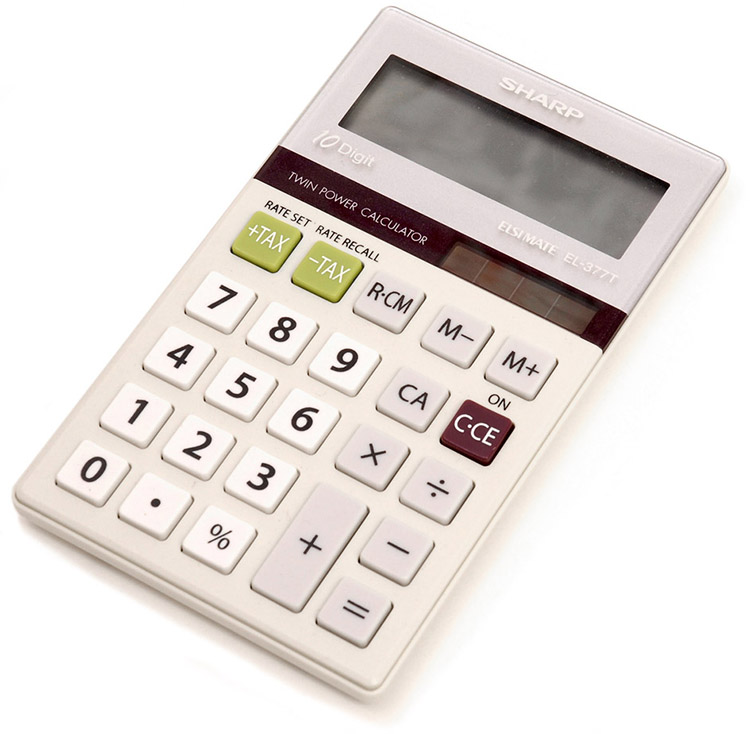# 20.4 Electric power and energy  (Page 4/7)

 Page 4 / 7

## Making connections: take-home experiment—electrical energy use inventory

1) Make a list of the power ratings on a range of appliances in your home or room. Explain why something like a toaster has a higher rating than a digital clock. Estimate the energy consumed by these appliances in an average day (by estimating their time of use). Some appliances might only state the operating current. If the household voltage is 120 V, then use $P=\text{IV}$ . 2) Check out the total wattage used in the rest rooms of your school’s floor or building. (You might need to assume the long fluorescent lights in use are rated at 32 W.) Suppose that the building was closed all weekend and that these lights were left on from 6 p.m. Friday until 8 a.m. Monday. What would this oversight cost? How about for an entire year of weekends?

## Section summary

• Electric power $P$ is the rate (in watts) that energy is supplied by a source or dissipated by a device.
• Three expressions for electrical power are
$P=\text{IV,}$
$P=\frac{{V}^{2}}{R}\text{,}$

and

$P={I}^{2}R\text{.}$
• The energy used by a device with a power $P$ over a time $t$ is $E=\text{Pt}$ .

## Conceptual questions

Why do incandescent lightbulbs grow dim late in their lives, particularly just before their filaments break?

The power dissipated in a resistor is given by $P={V}^{2}/R$ , which means power decreases if resistance increases. Yet this power is also given by $P={I}^{2}R$ , which means power increases if resistance increases. Explain why there is no contradiction here.

## Problem exercises

What is the power of a $1.00×{\text{10}}^{\text{2}}\phantom{\rule{0.25em}{0ex}}\text{MV}$ lightning bolt having a current of ${2.00 × 10}^{\text{4}}\phantom{\rule{0.25em}{0ex}}\text{A}$ ?

$2\text{.}\text{00}×{\text{10}}^{\text{12}}\phantom{\rule{0.25em}{0ex}}\text{W}$

What power is supplied to the starter motor of a large truck that draws 250 A of current from a 24.0-V battery hookup?

A charge of 4.00 C of charge passes through a pocket calculator’s solar cells in 4.00 h. What is the power output, given the calculator’s voltage output is 3.00 V? (See [link] .)The strip of solar cells just above the keys of this calculator convert light to electricity to supply its energy needs. (credit: Evan-Amos, Wikimedia Commons)

How many watts does a flashlight that has $6.00×{\text{10}}^{\text{2}}\phantom{\rule{0.25em}{0ex}}\text{C}$ pass through it in 0.500 h use if its voltage is 3.00 V?

Find the power dissipated in each of these extension cords: (a) an extension cord having a $0\text{.}\text{0600}\phantom{\rule{0.25em}{0ex}}\text{-}\phantom{\rule{0.25em}{0ex}}\Omega$ resistance and through which 5.00 A is flowing; (b) a cheaper cord utilizing thinner wire and with a resistance of $0\text{.}\text{300}\phantom{\rule{0.25em}{0ex}}\Omega .$

(a) 1.50 W

(b) 7.50 W

Verify that the units of a volt-ampere are watts, as implied by the equation $P=\text{IV}$ .

Show that the units $1\phantom{\rule{0.25em}{0ex}}{\text{V}}^{2}/\Omega =1\text{W}$ , as implied by the equation $P={V}^{2}/R$ .

$\frac{{V}^{2}}{\Omega }=\frac{{V}^{2}}{\text{V/A}}=\text{AV}=\left(\frac{C}{s}\right)\left(\frac{J}{C}\right)=\frac{J}{s}=1\phantom{\rule{0.25em}{0ex}}\text{W}$

Show that the units $1\phantom{\rule{0.25em}{0ex}}{\text{A}}^{2}\cdot \Omega =1\phantom{\rule{0.25em}{0ex}}\text{W}$ , as implied by the equation $P={I}^{2}R$ .

Verify the energy unit equivalence that $1\phantom{\rule{0.25em}{0ex}}\text{kW}\cdot \text{h = 3}\text{.}\text{60}×{\text{10}}^{6}\phantom{\rule{0.25em}{0ex}}\text{J}$ .

$1\phantom{\rule{0.25em}{0ex}}\text{kW}\cdot \text{h=}\left(\frac{1×{\text{10}}^{3}\phantom{\rule{0.25em}{0ex}}\text{J}}{\text{1 s}}\right)\left(1 h\right)\left(\frac{\text{3600 s}}{\text{1 h}}\right)=3\text{.}\text{60}×{\text{10}}^{6}\phantom{\rule{0.25em}{0ex}}\text{J}$

Electrons in an X-ray tube are accelerated through $1.00×{\text{10}}^{\text{2}}\phantom{\rule{0.25em}{0ex}}\text{kV}$ and directed toward a target to produce X-rays. Calculate the power of the electron beam in this tube if it has a current of 15.0 mA.

An electric water heater consumes 5.00 kW for 2.00 h per day. What is the cost of running it for one year if electricity costs $\text{12.0 cents}\text{/kW}\cdot \text{h}$ ? See [link] .On-demand electric hot water heater. Heat is supplied to water only when needed. (credit: aviddavid, Flickr)

\$438/y

What is the difference between a principle and a law
the law is universally proved. The principal depends on certain conditions.
Dr
what does the speedometer of a car measure ?
Car speedometer measures the rate of change of distance per unit time.
Moses
describe how a Michelson interferometer can be used to measure the index of refraction of a gas (including air)
using the law of reflection explain how powder takes the shine off a person's nose. what is the name of the optical effect?
WILLIAM
is higher resolution of microscope using red or blue light?.explain
WILLIAM
can sound wave in air be polarized?
Unlike transverse waves such as electromagnetic waves, longitudinal waves such as sound waves cannot be polarized. ... Since sound waves vibrate along their direction of propagation, they cannot be polarized
Astronomy
A proton moves at 7.50×107m/s perpendicular to a magnetic field. The field causes the proton to travel in a circular path of radius 0.800 m. What is the field strength?
derived dimenionsal formula
what is the difference between mass and weight
assume that a boy was born when his father was eighteen years.if the boy is thirteen years old now, how is his father in
Isru
what is airflow
derivative of first differential equation
why static friction is greater than Kinetic friction
draw magnetic field pattern for two wire carrying current in the same direction
An American traveler in New Zealand carries a transformer to convert New Zealand’s standard 240 V to 120 V so that she can use some small appliances on her trip.
What is the ratio of turns in the primary and secondary coils of her transformer?
nkombo
what is energy
Yusuf
How electric lines and equipotential surface are mutually perpendicular?
The potential difference between any two points on the surface is zero that implies È.Ŕ=0, Where R is the distance between two different points &E= Electric field intensity. From which we have cos þ =0, where þ is the angle between the directions of field and distance line, as E andR are zero. Thus
sorry..E and R are non zero...

#### Get Jobilize Job Search Mobile App in your pocket Now!ByByBy Yacoub JayoghliBy OpenStaxBy OpenStaxBy Madison ChristianBy OpenStaxBy OpenStaxByBy OpenStaxBy RhodesBy Jonathan Long